Home

# Compactness of Commutators for Singular Integrals on Morrey Spaces

## Abstract

In this paper we characterize the compactness of the commutator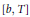$\left[ b,\,T \right]$ for the singular integral operator on the Morrey spaces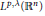${{L}^{p,\lambda }}\left( {{\mathbb{R}}^{n}} \right)$ . More precisely, we prove that if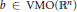$b\,\in \,\text{VMO}\left( {{\mathbb{R}}^{n}} \right)$ , the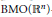$\text{BMO}\left( {{\mathbb{R}}^{n}} \right)$ -closure of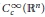$C_{c}^{\infty }\left( {{\mathbb{R}}^{n}} \right)$ , then$\left[ b,\,T \right]$ is a compact operator on the Morrey spaces${{L}^{p,\lambda }}\left( {{\mathbb{R}}^{n}} \right)$ for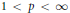$1\,<\,p\,<\,\infty$ and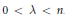$0\,<\,\lambda \,<\,n$ . Conversely, if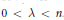$b\,\in \,\text{BMO}\left( {{\mathbb{R}}^{n}} \right)$ and$\left[ b,\,T \right]$ is a compact operator on the${{L}^{p,\lambda }}\left( {{\mathbb{R}}^{n}} \right)$ for some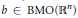$p\,\left( 1\,<\,p\,<\,\infty \right)$ , then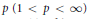$b\,\in \,\text{VMO}\left( {{\mathbb{R}}^{n}} \right)$ . Moreover, the boundedness of a rough singular integral operator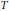$T$ and its commutator$\left[ b,\,T \right]$ on${{L}^{p,\lambda }}\left( {{\mathbb{R}}^{n}} \right)$ are also given. We obtain a sufficient condition for a subset in Morrey space to be a strongly pre-compact set, which has interest in its own right.

• # Send article to Kindle

Note you can select to send to either the @free.kindle.com or @kindle.com variations. ‘@free.kindle.com’ emails are free but can only be sent to your device when it is connected to wi-fi. ‘@kindle.com’ emails can be delivered even when you are not connected to wi-fi, but note that service fees apply.

Find out more about the Kindle Personal Document Service.

Compactness of Commutators for Singular Integrals on Morrey Spaces
Available formats
×

# Send article to Dropbox

To send this article to your Dropbox account, please select one or more formats and confirm that you agree to abide by our usage policies. If this is the first time you use this feature, you will be asked to authorise Cambridge Core to connect with your <service> account. Find out more about sending content to Dropbox.

Compactness of Commutators for Singular Integrals on Morrey Spaces
Available formats
×

# Send article to Google Drive

To send this article to your Google Drive account, please select one or more formats and confirm that you agree to abide by our usage policies. If this is the first time you use this feature, you will be asked to authorise Cambridge Core to connect with your <service> account. Find out more about sending content to Google Drive.

Compactness of Commutators for Singular Integrals on Morrey Spaces
Available formats
×

## References

Hide All
MathJax
MathJax is a JavaScript display engine for mathematics. For more information see http://www.mathjax.org.

# Compactness of Commutators for Singular Integrals on Morrey Spaces

## Metrics

### Full text viewsFull text views reflects the number of PDF downloads, PDFs sent to Google Drive, Dropbox and Kindle and HTML full text views.

Total number of HTML views: 0
Total number of PDF views: 0 *Loading metrics...

### Abstract viewsAbstract views reflect the number of visits to the article landing page.

Total abstract views: 0 *Loading metrics...

* Views captured on Cambridge Core between <date>. This data will be updated every 24 hours.

Usage data cannot currently be displayed